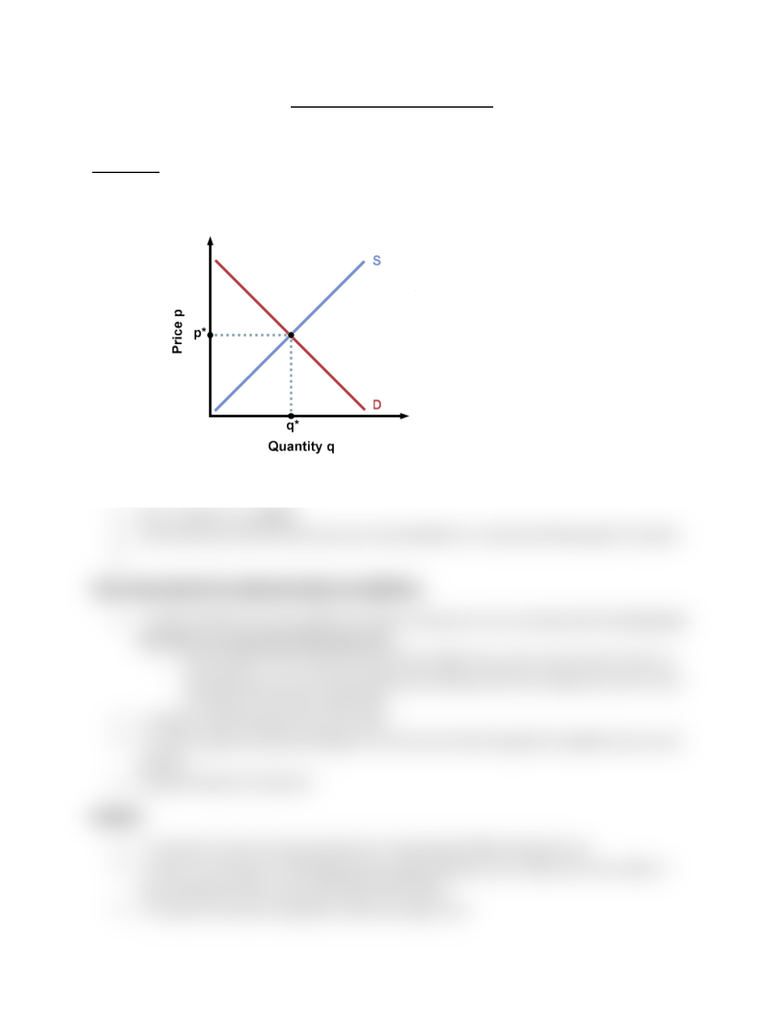Textbook Notes (280,000)
CA (160,000)
McMaster (10,000)
ECON (800)
ECON 1BB3 (300)
Chapter 4

ECON 1BB3 Chapter Notes - Chapter 4: Pencil, Demand Curve, Normal Good

Department
Economics
Course Code
ECON 1BB3
Professor
Bridget O' Shaughnessy
Chapter
4

This preview shows half of the first page. to view the full 2 pages of the document.Richard Damra Monday, January 21, 2013
Econ 1BB3 Chapter 4 Equilibrium
Equilibrium
Equilibrium price: the price for which Qs = Qd
Equilibrium quantity: the quantity that corresponds to equilibrium price
Comparative Statics: We start at equilibrium, something will change one of the curves will shift
and we end up with new eq. so we compare old equilibrium with new equilibrium.
If Qs > Qd there is a surplus
Stocks build up and firms decrease price until equilibrium is restored (include graph of surplus)
Three step program for analyzing changes in equilibrium
1. Decide whether the event shifts the supply or demand curve (or perhaps both). It is very rare
that there is an event that shifts both curve.
o Ask yourself: If I am a buyer does this shock affect how much I want to buy? If yes it is
demand then, Say I am a firm producing something, does this change how much I want
to produce? If yes then supply shift
2. Decide in which direction the curve shifts
3. Use the supply-and-demand diagram to see how the shift changed the equilibrium price and
quantity.
Example: Market for HB pencil
Example
1. Consumer income increases (pencils are normal goods) Affect demand curve
2. There is an increase in standardized tests, affects demand curve. When one curve shifts, it
moves along the other curves, DOES NOT SHIFT BOTH.
3. Increase in the price of graphite, shifts the supply curve
You're Reading a Preview

Unlock to view full version